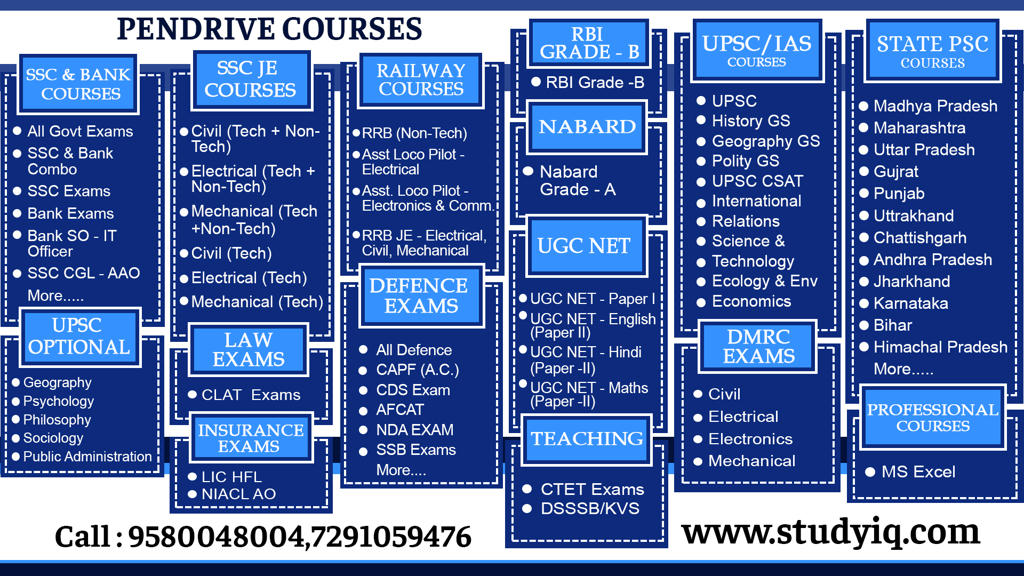# Time & Distance Questions – Part 1 – Maths & Reasoning | PDF DownloadQ1. A train operates with a speed of 72 km / h. What his speed in m/sec?

1. 20m / sec
2. 25m / sec
3. 30m / sec
4. 40m / sec

Q2. One train runs at 15 m / sec. what his speed in km / hour?

1. 50 km / h
2. 52 km / h
3. 54 km / h
4. 56 km / h

Q3. 360m Long train running at a speed of 36 m/sec How long will it cross the telephone pillar?

1. 20 Sec.
2. 15 Sec.
3. 17 Sec.
4. 10 Sec.

Q4. If a 100 m long train is running at a speed of 20 km / h, how long will it take to cross a person standing on the platform?

1. 15 Sec.
2. 20 Sec.
3. 18 Sec.
4. 25 Sec.

Q5. A train whose length is 125 m If it crosses of any tree standing on the edge of the railway line in 30 sec then what is the speed of the train?

1. 14 Km/h
2. 15 Km/h
3. 12 Km/h
4. 16 Km/h

Q6. 250 m long train crosses A person standing on a train platform in 25 sec What is the speed of the train?

1. 25 Km/h
2. 30 km/h
3. 36 Km/h
4. 40 Km/h

Q7. A train crosses a telephonic pillar in 30 seconds while walking with a speed of 54 km / hour , what is the length of the train?

1. 400 m
2. 420 m
3. 430 m
4. 450 m

Q8. A train running at 36 km / hr crosses a person standing on platform in 10 sec. What is the length of the train?

1. 120 m
2. 100 m
3. 150 m
4. 200 m

Q9. Trains of two equal lengths are running on parallel tracks in the opposite direction at a speed of 65 km / hr and 85 km / h respectively. They cross each other in 6 seconds. Accordingly, what is the length of each car?

1. 110 m
2. 115 m
3. 125 m
4. 150 m

Q10. Two rugs of 180 m and 220 m are running in the opposite directions at a speed of 40 km / hr and 50 km / hr respectively, how often will they cross each other?

1. 17 Sec.
2. 20 Sec.
3. 16 Sec.
4. 18 Sec.

Q11. A train runs at 60 km / h and crosses the platform in C seconds. If the length of the train is 200 m and the length of the platform is 300m then what is the value of C?

1. 20 Sec.
2. 30 Sec.
3. 40 Sec.
4. 50 Sec

Q12. A 270 meter long train is running at a speed of 36 km / h. Accordingly in how much time will he cross the 180 m long bridge?

1. 30 Sec.
2. 45 Sec.
3. 50 Sec.
4. 35 Sec

Q13. A 160 meter long train crosses a 320 meter tall platform in 36 seconds, then what is the train’s speed?

1. 40 Km/h
2. 45 km/h
3. 60 Km/h
4. 48 Km/h

Q14. 130 meter long train runs a bridge with a speed of 45 km / hour and crosses 30 seconds, then find the length of the bridge-

1. 115 m
2. 120 m
3. 145 m
4. 250 m

Q15. A train crosses one pillar of telegraph and a bridge of 264 m in 8 seconds and 20 seconds, respectively. So what is the speed of the train?

1. 69.5 Km/h
2. 70 Km/h
3. 79 km/h
4. 79.2 Km/h

Q16. A train crosses 50m long platform in 14 seconds and man crosses the platform in 10 seconds. What is the train speed?

24 Km/h

36 Km/h

40 Km/h

45 Km/h

Q17. A train crosses 108 meter long and crosses another 164 m long station in 16 seconds in 12 seconds. So what’s the length of the train?

1. 64 m
2. 60 m
3. 80 m
4. 84 m

Q18. 240 meter long train crosses one pillar in 24 seconds. How long will the train cross the 650m long platform?

1. 89 Sec.
2. 50 Sec.
3. 100 Sec.
4. 150 Sec.